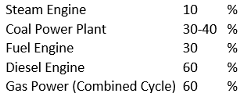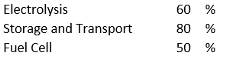## Hydrogen

Hydrogen can be produced from water by electrolysis. In this process, water is broken down into its components hydrogen and oxygen by means of an electric current. The hydrogen can then in turn be used to generate energy by burning it with oxygen. If renewable electricity is used for production, then this hydrogen cycle is climate neutral.

This all sounds very simple and plausible, however, physics stands in the way of an economic application.

In any process where energy is converted to another form of energy, there are losses. The essential conversion of energy is the conversion of heat into work. The fundamental work on this was published by Sidi Carnot in 1824, almost 200 years ago.

A crucial parameter in the conversion of heat into work is the efficiency. This is the ratio of the work gained to the energy used.

η = w / q

The efficiencies of various machines for converting heat into work are, for example:The efficiency results from the temperature differences that occur during a process. The diesel engine has a very high efficiency due to the very high combustion temperature. The first steam engine, on the other hand, had an efficiency of only 1%.

The hydrogen economy involves the following processes with corresponding efficiencies:This results in an overall efficiency of 0.6 x 0.8 x 0.5 = 0.24

This efficiency is the maximum achievable. In practice, this will be significantly lower at approx. 10 - 15 %.

A derivation of these efficiencies can be found here.